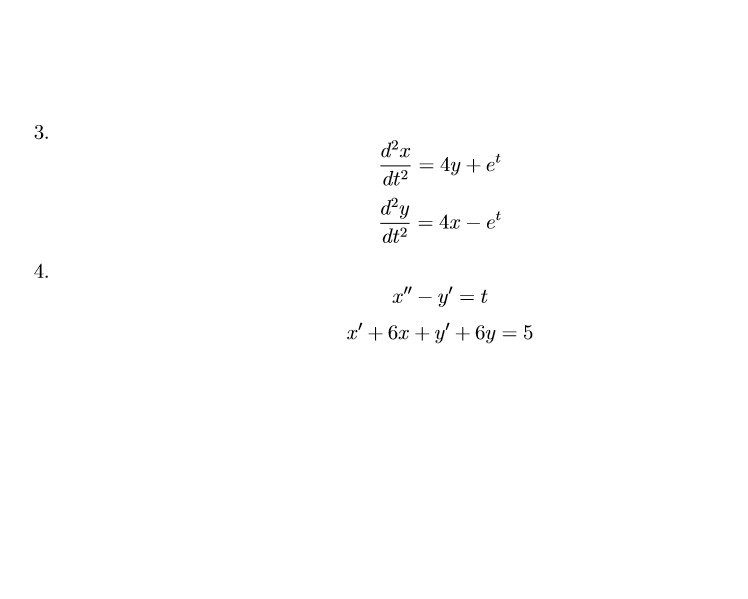# Solved: Solve The Given System Of DEs By Systematic Elimination. Assume The Independent Variable Is T. Pls Write The Solution In Detail, I Want To Figure Out How To Do This Kind Of Question Instead Of

By |

Solve the given system of DEs by systematic elimination. Assume the independent variable is t.

pls write the solution in detail, I want to figure out how to do this kind of question instead of just knowing the answer3. 4y e dt2 d2y et dt2 4 " - y/ = t x6 y+ 6y = 5